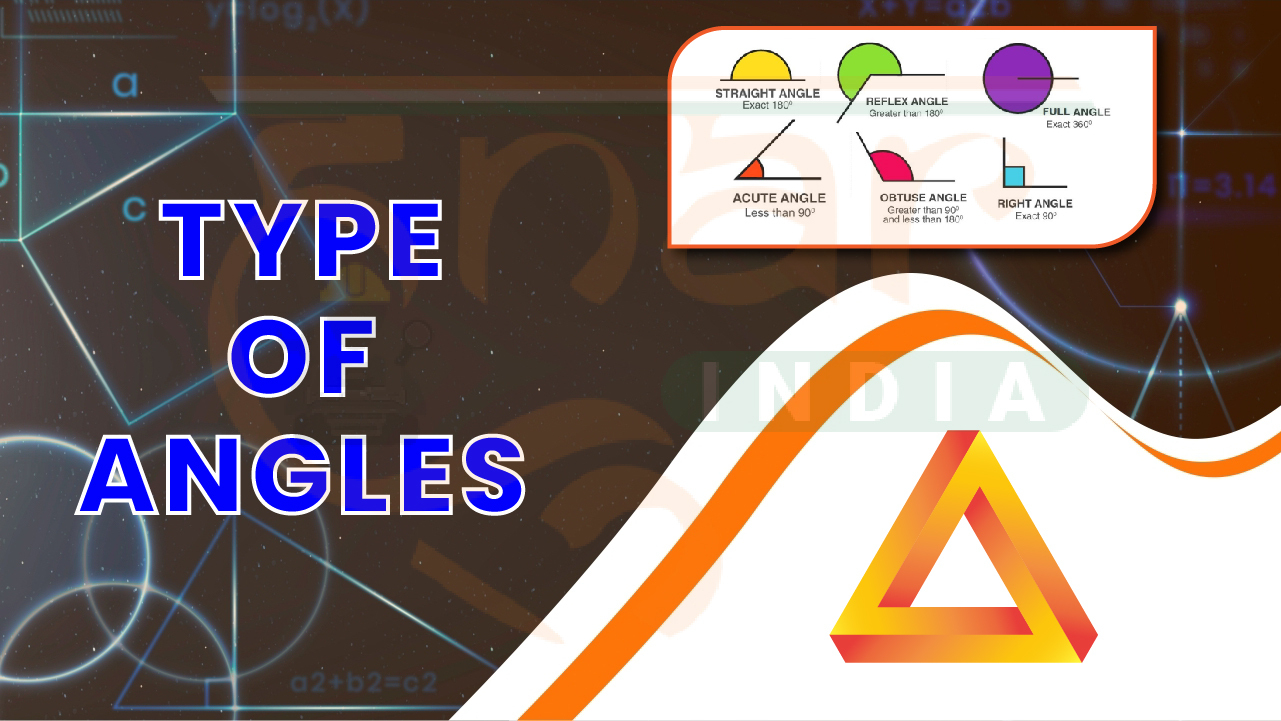×
Type of Angles

# Type of Angles

#### Tags# Type of Angles

Brief description of each type of angle:

1. Acute Angle: A small angle that measures less than 90 degrees.
2. Right Angle: An angle that measures exactly 90 degrees, forming an "L" shape.
3. Obtuse Angle: An angle that is larger than 90 degrees but smaller than 180 degrees.
4. Straight Angle: An angle that measures exactly 180 degrees, forming a straight line.
5. Reflex Angle: An angle that is larger than 180 degrees but smaller than 360 degrees.
6. Complementary Angles: Two angles that add up to 90 degrees.
7. Supplementary Angles: Two angles that add up to 180 degrees.
8. Adjacent Angles: Two angles that share a common vertex and side.
9. Vertical Angles: Opposite angles formed by the intersection of two lines, with equal measures.
10. Corresponding Angles: Angles in the same relative position when a transversal intersects two lines, with equal measures.
11. Alternate Interior Angles: Interior angles on opposite sides of the transversal, with equal measures.
12. Alternate Exterior Angles: Exterior angles on opposite sides of the transversal, with equal measures.

These descriptions provide a quick overview of each angle type.Types of Angles.pdf
##### Magical Rule for Number System Part 01

We don't support landscape mode yet. Please go back to Portrait mode for the best experience.

# Hunar India

Typically replies within a day

Hi there